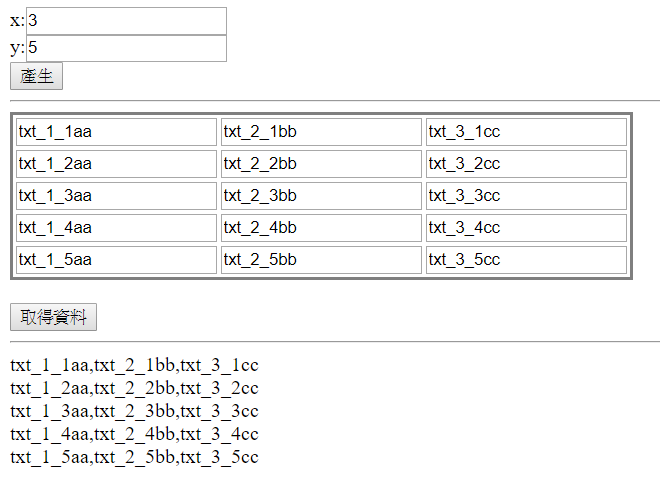### 一些觀念的共享：

#### 如何保留狀態：

Web是沒有狀態的，為了保留狀態，在WebForm中，會藉助於『ViewState』來協助保留狀態。然而，動態產生控制項，產生了，如何保留這些控制項，還有控制項的狀態。最簡單的方式，就是把動態產生控制項的動作，寫在Page的Init事件。

### 範例：

PostBack後，如果資料與上次的X,Y不同，會重新再次產生依據X,Y的數字，產生陣列型的TextBox在Table1控制項中（如果相同，就不再重新產生）aspx:

x:<asp:TextBox ID="txtX" runat="server"></asp:TextBox><br />
y:<asp:TextBox ID="txtY" runat="server"></asp:TextBox><br />
<asp:Button ID="btnGenArrayList" runat="server" Text="產生" />
<hr />
<asp:Table ID="Table1" runat="server" BorderStyle="Solid">
</asp:Table>
<br />
<asp:Button ID="btnGetDatas" runat="server" Text="取得資料" style="margin-bottom: 0px" />
<hr />
<asp:Label ID="lblRlt" runat="server" Text=""></asp:Label>

CodeFile(aspx.vb):

    Private Sub tDynamicArrayListGV_Init(sender As Object, e As EventArgs) Handles Me.Init
If IsPostBack Then
Dim ReGen As Boolean = False
Dim X As Integer = 0
If IsNumeric(Request.Form("txtX")) Then
X = CInt(Request.Form("txtX"))
If X <> ViewState("X") Then
ReGen = True
ViewState("X") = X
End If

End If
Dim Y As Integer = 0
If IsNumeric(Request.Form("txtY")) Then
Y = CInt(Request.Form("txtY"))
If Y <> ViewState("Y") Then
ReGen = True
ViewState("Y") = Y
End If
End If
If ReGen And X > 0 And Y > 0 Then
Dim tCell As TableCell
Dim tRow As TableRow
Dim tTxt As TextBox
For y1 = 1 To Y
tRow = New TableRow()
For x1 = 1 To X
tCell = New TableCell
tTxt = New TextBox()
tTxt.ID = "txt_" & x1.ToString & "_" & y1.ToString
tTxt.Text = "txt_" & x1.ToString & "_" & y1.ToString
Next
Next
End If
End If
End Sub

Protected Sub btnGetDatas_Click(sender As Object, e As EventArgs) Handles btnGetDatas.Click
Dim X As Integer = ViewState("X")
Dim Y As Integer = ViewState("Y")

Dim Rlt As String = ""
Dim tTxt As TextBox
For y1 = 1 To Y
For x1 = 1 To X
tTxt = CType(Me.Table1.FindControl("txt_" & x1.ToString & "_" & y1.ToString), TextBox)
If tTxt IsNot Nothing Then
Rlt &= tTxt.Text & ","
End If
Next
Rlt = Mid(Rlt, 1, Len(Rlt) - 1) & "<br/>"
Next
Me.lblRlt.Text = Rlt
End Sub

1. 寫在Page_Init事件中：
動態產生控制項，重點在於要把產生控制項的過程，寫在Page_Init事件中，這樣ViewState的作用就自然而生，不必特別為了保留這些動態產生控制項的狀態，特別寫什麼。
2. Page_Init中，用Request.Form取得送過來資料：
不過由於寫在Page_Init事件中，無法用『Me.TextBox1.Text』取得畫面輸入的內容，所以要改由『Request.Form』來取得。

• 歡迎轉貼本站的文章，不過請在貼文主旨上加上【轉貼】，並在文章中附上本篇的超連結與站名【topcat姍舞之間的極度凝聚】，感恩大家的配合。
• 小喵大部分的文章會以小喵熟悉的語言VB.NET撰寫，如果您需要C#的Code，也許您可以試著用線上的工具進行轉換，這裡提供幾個參考

 Microsoft MVP Visual Studio and Development Technologies (2005~2019/6)topcat Blog:http://www.dotblogs.com.tw/topcat